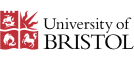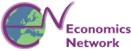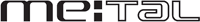# Algebra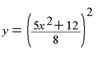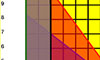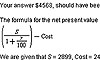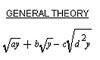Students and teaching staff can download a fairly extensive set of tests to try out at home. The following file offers a number of question sets which are re-realised each time they are used. It therefore provides many millions of potential economics problems to solve. Download instructions are at the bottom of the page.

These questions can also be used through the MathsEG online interface.

This file contains the following question sets

• Algebra diagnostic test 1
• Algebra diagnostic test 2
• Algebraic functions
• Completing the square
• Expanding brackets
• Indices
• Linear equations
• Modelling
• Partial fractions
• Pascal's triangle
• Proportionality
• Rational functions simplification
• Rearranging equations
• Roots and factors
• Simultaneous equations
• Summations
• Terminology and expressions

These are listed alphabetically; you should not necessarily do the tests in the order detailed above.

• Install Adobe's SVG viewer plug-in once only.
• Ensure you also have Java.